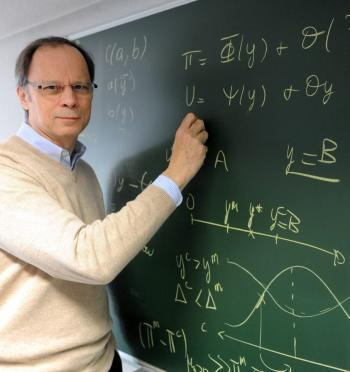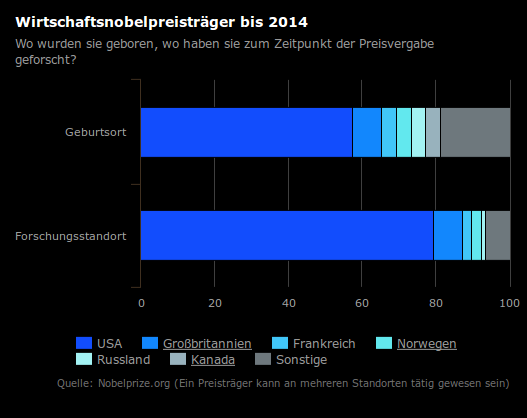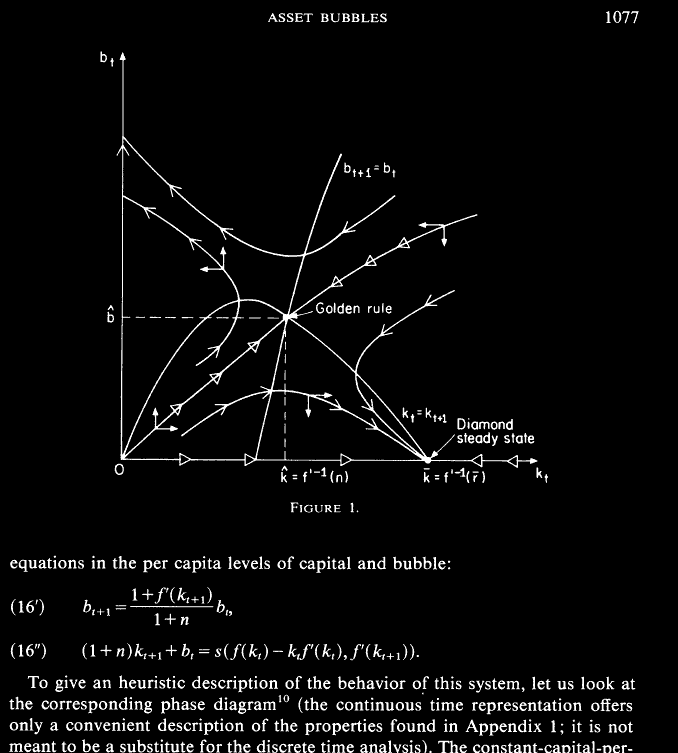The 2014 Nobel prize in Economics went to the French economist Jean Tirole who worked on a theory of market failures. Summarizing in one sentence, Tirole showed that market regulation is complex and that rules should be adapted to each situation. His work contains quite a bit of mathematics on the 21a and 21b level. Here is an example of a paper on Asset bubbles [PDF]. a topic which became relevant recently. How do bubbles form and aggregate? Which situations lead to bubbles and what is the nature and the consequences of bubbles? Here is a more recent paper on liquidity [PDF]. The mathematics of these papers deals more with discrete dynamical systems. given by vector fields F(x,y) = . One is first of all interested in places, where the vector field is <0,0>. These are called equilibrium points. Then one is interested in the stability of the equilibria and the dynamics X= -> F(x,y). We will mostly deal in 21a with situations where F(x,y) is the gradient of some functions f. The equilibria of F are the critical points of f. Working in smaller dimensions and simple looking models whose understanding can have enormous consequences is typical.